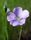## Homework: PROC GLM

The GLM Procedure

Repeated Measures Analysis of Variance

Analysis of Variance of Contrast Variables

Tests of Hypotheses for Between Subjects Effects

Source             DF       Type 111 SS               Mean Square               F Value           Pr > F

Group              1          5.09336806                 5.09336806                 6.12                 0.0216

Error                22        18.31139722               0.83233624

The GLM Procedure

Repeated Measures Analysis of Variance

Univariate Tests of Hypotheses for within Subjects Effects

Source             DF       Type 111 SS   Mean Square   F Value Pr > F            G – G              H - F

Time    1          2          2.21393611     1.10696806     5.55     0.0071            0.0130             0.0103

Time*Group    2          2.06266944     0.32958182     5.17     0.0096             0.0167             0.0135

Error (time)     44        8.77452778     0.19942109

Greenhouse-Geisser Epsilon  0.7768

Huynh-Feldt Epsilon               0.8628

1. What is the value for the sums-of-squares that is obtained by finding deviations of the treatment means from the grand mean. ? (Use two-digit decimal)
2. What is the value for SSwithin
3. What is the value for the sums-of-squares that is obtained by finding deviations of the means at each point in time and the grand mean? (Use two-digit decimal)
4. What is the F value that is obtained from MStime / MSresidual?LinusH
Tourmaline | Level 20

## Re: Homework: PROC GLM

I renamed the thread to better describe the subject.

Data never sleeps
Discussion stats